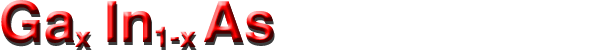## Band structure and carrier concentration

Basic Parameters
Band structure
Intrinsic carrier concentration
Lasing wavelength
Effective Density of States in the Conduction and Valence Band
Temperature Dependences
Dependences on Hydrostatic Pressure
Band Discontinuities at Heterointerfaces
Energy gap narrowing at high doping levels
Effective Masses and Density of States
Donors and Acceptors

### Basic Parameters for GaxIn1-xAsyP1-y

##### Zinc Blende crystal structure
 Ga0.47In0.53As GaxIn1-xAs Remarks Referens Energy gaps, Eg 0.74 eV (0.36+0.63x+0.43x2) eV 300 K Goetz et al.(1983) Energy gaps, Eg (0.4105+0.6337x+0.475x2) eV 2 K Goetz et al.(1983) Electron affinity 4.5 eV (4.9-0.83x) eV 300 K Conduction band Energy separation between X valley and top of the valence band EX 1.33 eV (1.37-0.63x+1.16x2) eV 300 K Goetz et al.(1983) Energy separation between L valley and top of the valence band EL 1.2 eV (1.08-0.02x+0.65x2) eV 300 K Goetz et al.(1983) Effective conduction band density of states 2.1·1017 cm-3 see Temerature dependences Valence band Energy separation of spin-orbital splitting Eso *** *** Effective valence band density of states 7.7·1018 cm-3 see Temerature dependences Intrinsic carrier concentration 6.3·1011 cm-3 see Temerature dependences

### Band structure for GaxIn1-xAs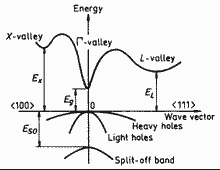GaxIn1-xAs (zinc blende, cubic). Band structure Important minima of the conduction band and maxima of the valence band.. For details see Goldberg Yu.A. & N.M. Schmidt (1999) .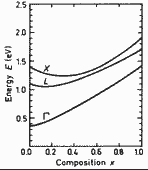GaxIn1-xAs. Energy gap Eg Energy separations between Γ- ,X-, and L -conduction band minima and top of the valence band vs. composition parameter x. Porod and Ferry (1983)

Interfacial elastic strain induced by lattice parameter mismatch between epilayer and substrate results in significant band-gap shifts: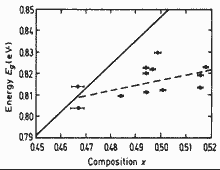GaxIn1-xAs. Energy band gap Eg of unstrained (solid line) and strained (dashed line and experimental points) vs. composition parameter x. Solid line is calculated according to Eg= (0.4105+0.6337x+0.475x2) eV. Experimental points are obtained at 4K. Kuo et al.(1985)Brillouin zone of the face centered cubic lattice, the Bravais lattice of the diamond and zincblende structures.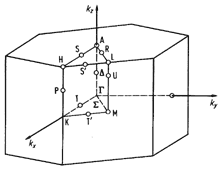Brillouin zone of the hexagonal lattice.

### Temperature Dependences

 Eg (x,T)= 0.42 + 0.625x -[5.8/(T+300)-4.19 /(T+271)]·10-4T2x-                   - 4.19·10-4T2/(T+271) +0.475x2 (eV) (eV) GaxIn1-xAs Paul et al.(1991) Eg (x,T)= Eg (0) + (6x2- 8.6x +5.2)·10-4 T2/(337x2- 455x +196) GaxIn1-xAs on Ga As Karachevtseva et al.(1994) Eg (x,T)= 0.42 + 0.625x -[5.8/(T+300)-4.19 /(T+271)]·10-4T2x-                   - 4.19·10-4T2/(T+271) +0.475x2 (eV) (eV) GaxIn1-xAs where T is temperature in degrees K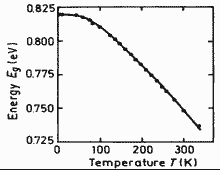Ga0.47In0.53As. Energy gap Eg of vs. temperature Points are experimental data. Solid line is theoretical calculation. Eg(0)=821.5 ± 0.2 meV. Zielinski et al.(1986)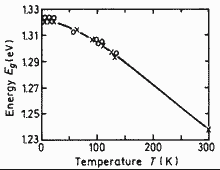Ga0.87In0.13As. Energy gap Eg of vs. temperature Points are experimental data. Solid line -- 1.321 - 4.1·10-4 T2/(T+139) Karachevtseva et al.(1994)

### Intrinsic carrier concentration:

ni = (Nc·Nv)1/2exp(-Eg/(2kBT)) ~= 4.82 x 1015 · [(0.41-0.09x)3/2 +(0.027+0.047x)3/2]1/2 x
x(0.025+0.043x)3/4[ T3/2 exp(-ν/2)(1+3.75/ν +3.28/ν2 -2.46/ν3)1/2 ] (cm-3) ,
where ν=E(x,T)/2kT
Paul et al.(1991).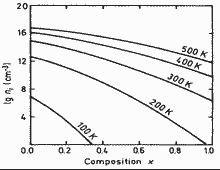GaxIn1-xAs. Intrinsic carrier concentration vs. temperature for GaxIn1-xAs. T = 100K; 200K; 300K; 400K; 500K; Paul et al.(1991)
ni = 6.3x1011 cm-3 for Ga0.47In0.53As at 300K

### Effective density of states in the conduction band: Nc

Nc ~= 4.82 x 1015 · (mΓ/m0)3/2T3/2 (cm-3) ~= 4.82 x 1015 · (0.023+0.037x+0.003x2)3/2 T3/2 (cm-3) :

### Effective density of states in the valence band: Nv

Nv ~= 4.82 x 1015 · (mh/m0)3/2 T3/2 (cm-3)= 4.82 x 1015 · (0.41-0.1x)3/2 T3/2 (cm-3) :

### Dependence on Hydrostatic Pressure

 Eg (0.47,P)~= (0.796+10.9x 10-3 ·P -30x10-6 ·P2) eV 80K, Ga0.47In0.53As x=0.47 Lambkin and Dunstan (1988) Eg (0.47,P)~= (0.733+11.0x 10-3 ·P -27x10-6 ·P2) eV 300K, Ga0.47In0.53As x=0.47 Eg (0.0, P)~= (Eg (0)+4.8x 10-3 ·P) eV 300K, InAs x=0. Eg (1.0, P)~= (Eg (0)+12.6x 10-3 ·P -37.7x10-6 ·P2) eV 300K, GaAs x=1.
where P is pressure in kbar.

### Energy gap narrowing at high doping levels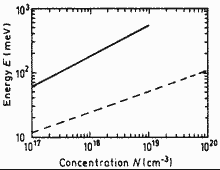Ga0.47In0.53As. Energy gap narrowing Eg vs. donor (solid line) and acceptor (dashed line) doping density solid line -- donor doping density; dashed line -- acceptor doping density Jain et al. (1990)
 ΔEg ~= (A ·N1/3 10-9 +B ·N1/4 10-7 +C ·N1/2 10-12) meV 300K, Ga0.47In0.53As x=0.47 where n : A=15.5;   B=1.95;   C=159 300K, Ga0.47In0.53As x=0.47 p : A= 9.2;   B=3.57;   C=3.65 300K, Ga0.47In0.53As x=0.47 N -- carrier concentration in cm-3

### Band Discontinuities at Heterointerfaces

Band discontinuities at GaxIn1-xAs/AlyGa1-yAs heterointerface Shur (1990).
 Referens Conduction band discontinuity ΔEv =(ΔEg -ΔEv) eV Shur (1990) Valence band discontinuity ΔEc = (0.44 ΔEgg) eV Shur (1990) where ΔEgg (eV) = [1.247y + 1.5(1-x) - 0.4(1-x)2] (eV) is the difference       between Γ-valleys in GaxIn1-xAs and AlyGA1-yAs . Energy gap Eg discontinuity : ΔEg = ΔEgg for y<0.45 Energy gap Eg discontinuity : ΔEg = 0.476 +0.125y + 0.143y2 +1.5(1-x) - 0.4(1-x)2 for y>0.45 Band discontinuities ΔEv ~= 0.38 eV ΔEc ~= 0.22 eV at Ga0.47In0.53As/InP heterointerface Adachi (1992); Hybertsen (1991) Band discontinuities ΔEv ~= 0.2 eV ΔEc ~= 0.52 eV at Ga0.47In0.53As/Al0.48In0.52As heterointerface Adachi (1992); Hybertsen (1991) ΔEc /ΔEg = [0.653 + 0.1(1-x)] eV at GaxIn1-xAs/AlxIn1-xAs heterointerface Wolak et al.(1991)

### Effective Masses and Density of States:

#### Electrons

For wurtzite crystal structure the surfaces of equal energy in Γ valley should be ellipsoids, but effective masses in z direction and perpendicular directions are estimated to be approximately the same:
 Effective Electron Masses Remarks Referens Effective electron mass me= mΓ 0.023 -0.037x +0.003x2 mo GaxIn1-xAs; 300K; for Γ - valley Goldberg Yu.A. & N.M. Schmidt (1999) Effective electron mass me mΓ= 0.041 mo at n= 2x·1017 cm-3 mΓ= 0.074 mo at n= 6x·1018 cm-3 Ga0.47In0.53As; x=0.47 Pearsall (1982) mL = 0.29 mo ; ( L - valley ) mX = 0.68 mo ; ( X - valley ) Ga0.47In0.53As; x=0.47 Pearsall (1982)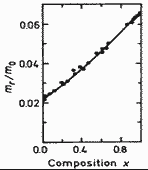GaxIn1-xAs. Electron effective mass vs. concentration x for GaxIn1-xAs; 300K Adachi (1992)

#### Holes

 Effective Masses for Zinc Blende GaN Remarks Referens Effective hole masses (heavy) mh mh ~= (0.41 -0.1x) mo GaxIn1-xAs; 300K; Goldberg Yu.A. & N.M. Schmidt (1999) Effective hole masses (light) mlp mlp ~= (0.026 -0.056x ) mo GaxIn1-xAs; 300K; Effective hole masses (split-off band) ms mso ~= 0.15 mo GaxIn1-xAs; 300K;

### Donors and Acceptors

 Ionization energies of Shallow Donors Remarks Sn, Ge, Si, C ~ 5 meV Ga0.47In0.53As; x=0.47 Goldberg Yu.A. & N.M. Schmidt (1999) Sn, Ge, Si, S, Se, Te > 1 meV InAs; x=0 Sn, Ge, Si, S, Se, Te ~ 6 meV GaAs; x=1 Ionization energies of Shallow Acceptor Mg ~ 25 meV Ga0.47In0.53As; x=0.47 Goldberg Yu.A. & N.M. Schmidt (1999) Zn ~ 20 meV Ga0.47In0.53As; x=0.47 Cd ~ 30 meV Ga0.47In0.53As; x=0.47 Mn ~ 50 meV Ga0.47In0.53As; x=0.47 Fe ~ 150 meV Ga0.47In0.53As; x=0.47 (above valence band), 280, 370, and 440 below conduction band Mg ~ 25 meV GaxIn1-xAs; 0